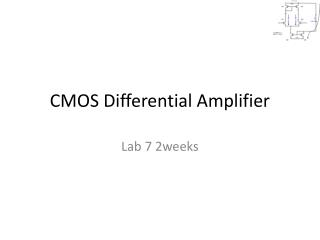DownloadDownload PresentationCMOS Differential Amplifier

# CMOS Differential Amplifier

Télécharger la présentation## CMOS Differential Amplifier

- - - - - - - - - - - - - - - - - - - - - - - - - - - E N D - - - - - - - - - - - - - - - - - - - - - - - - - - -
##### Presentation Transcript

1. CMOS Differential Amplifier Lab 7 2weeks

2. Diff Amp PMOS Current Mirror Diff pair Bias Current NMOS Current Mirror SPICE Models: NMOS LEVEL=2 +VTO=-0.7 KP=5000E-6 LAMBDA=0.02 GAMMA=0.45 +TOX=90E-9 NSUB=3.7E15 PMOS LEVEL=2 +VTO=0.7 KP=400E-6 LAMBDA=0.05 GAMMA=0.9 +TOX=90E-9 NSUB=3.7E15

3. Note Current Mirroring (Copy) ; ; Square Law Operation– Observations

4. Note CurreM4nt Mirroring (Copy) ; ; Square Law Operation– Observations

5. Developing the Bias Current • Setting biasing Current of Diff Amp, Note: • I R = ID6 • VDD -VSS= VSG + IB RB; R = (ß·2VDD - VTN) - (2·I))/(I·ß)

6. Diff Amp Current Behavior Starting Eq 1 & 2 Solving 1 & 2

7. Diff Amp Testing Case 1 Vin = 0, Io = 0 Case 2 -2xV < Vin < +2xV Case 3 Vin > | 2xV|; Io = IB

8. Diff Amp Voltage Behavior

9. Diff Amp Voltage Behavior